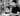GP

# Conditions Are Power-Law Distributed - A JS Example

I read Kent Beck's Conditions Are Power-Law Distributed: An Example today, and it made perfect sense. In a codebase there are lots of conditions used once, and few conditions used many times, the distribution following a power law.

Kent had some Bash code that he wrote to extract the distributions, but written for Python ifs, and I needed to adjust it to our Javascript/Typescript codebase (Currently of 304K lines):

``````\$ grep -R --include='*.js' 'if ' app | \
perl -nle 'print \$1 if /.*if\s*\((.*)\) /' | \
sort | uniq -c | sort -n -r | \
awk '{ print \$1}' | sort -n | uniq -c
2696 1
327 2
85 3
42 4
22 5
8 6
8 7
3 8
5 9
2 10
4 12
1 13
4 15
2 19
2 21
1 22
1 33
1 35
1 155``````

My goto tool for plotting is R (and R Studio) so let's output to a text file named `data.txt`.

Opening it in R Studio I ran the following code (And isn't R amazing?)

``````library(ggplot2)

data <- read.table("data.txt", col.names = c("Count", "Appearances"))
ggplot(data, aes(Appearances, Count)) + geom_point() +
scale_x_continuous(trans='log2') + scale_y_continuous(trans='log2')``````

This already adjusts the scales to be log2 based and we get a very similar power law distribution to Kent Beck's results.

Awesome!

Suggest an edit to this post